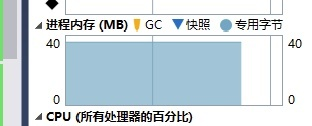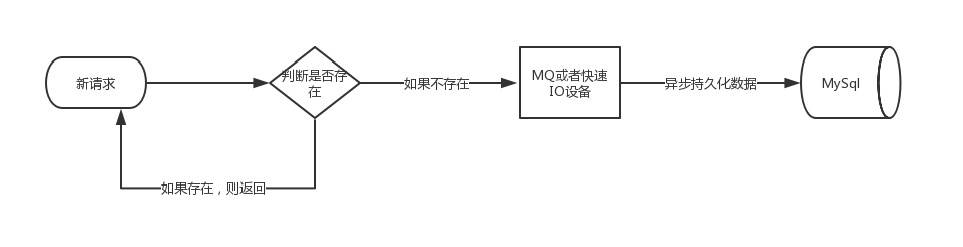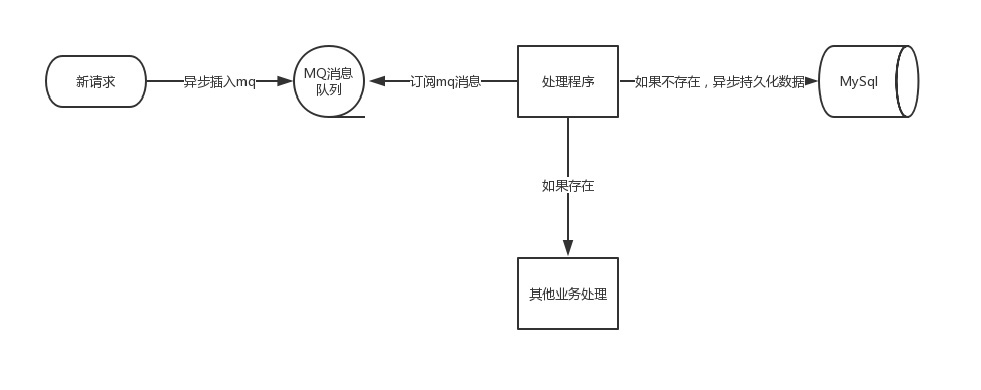# 优雅快速的统计千万级别 uv### 定义

﻿

PV是page view的缩写，即页面浏览量，通常是衡量一个网络新闻频道或网站甚至一条网络新闻的主要指标。网页浏览数是评价网站流量最常用的指标之一，简称为PV

﻿

UV是unique visitor的简写，是指通过互联网访问、浏览这个网页的自然人。

﻿

﻿

pv相比较uv来说，技术上比较容易一些，今天咱们就来说一说uv的统计，为什么说uv的统计相对来说比较难呢，因为uv涉及到同一个标准下的自然人的去重，尤其是一个uv千万级别的网站，设计一个好的uv统计系统也许并非想象的那么容易。

﻿

﻿

﻿

### 基于DB方案

﻿

﻿

﻿

if exists( select 1 from table where ip='ip' and dayid=dayid )　　Begin　　　　return 0　　Endelse　　Begin 　　　 insert into table .......　　End﻿

﻿

### 优化方案

﻿

﻿

1. 每次请求都需要判断是否已经存在相同的uv记录

2. 持久化uv数据不能影响正常的业务

3. uv数据的准确性可以忍受一定程度的误差

##### 优化哈希表

﻿

﻿

﻿

 class BloomFilter    {        BitArray container = null;      public BloomFilter(int length)        {            container = new BitArray(length);        }﻿        public void Set(string key)        {            var h1 = Hash1(key);            var h2 = Hash2(key);            var h3 = Hash3(key);            var h4 = Hash4(key);            container[h1] = true;            container[h2] = true;            container[h3] = true;            container[h4] = true;                    }        public bool Get(string key)        {            var h1 = Hash1(key);            var h2 = Hash2(key);            var h3 = Hash3(key);            var h4 = Hash4(key);﻿            return container[h1] && container[h2] && container[h3] && container[h4];        }﻿        //模拟哈希函数1         int Hash1(string key)        {            int hash = 5381;            int i;            int count;            char[] bitarray = key.ToCharArray();            count = bitarray.Length;            while (count > 0)            {                hash += (hash << 5) + (bitarray[bitarray.Length - count]);                count--;            }            return (hash & 0x7FFFFFFF) % container.Length;﻿        }         int Hash2(string key)        {            int seed = 131; // 31 131 1313 13131 131313 etc..            int hash = 0;            int count;            char[] bitarray = (key+"key2").ToCharArray();            count = bitarray.Length;            while (count > 0)            {                hash = hash * seed + (bitarray[bitarray.Length - count]);                count--;            }﻿            return (hash & 0x7FFFFFFF)% container.Length;        }         int Hash3(string key)        {            int hash = 0;            int i;            int count;            char[] bitarray = (key + "keykey3").ToCharArray();            count = bitarray.Length;            for (i = 0; i < count; i++)            {                if ((i & 1) == 0)                {                    hash ^= ((hash << 7) ^ (bitarray[i]) ^ (hash >> 3));                }                else                {                    hash ^= (~((hash << 11) ^ (bitarray[i]) ^ (hash >> 5)));                }                count--;            }﻿            return (hash & 0x7FFFFFFF) % container.Length;﻿        }        int Hash4(string key)        {            int hash = 5381;            int i;            int count;            char[] bitarray = (key + "keykeyke4").ToCharArray();            count = bitarray.Length;            while (count > 0)            {                hash += (hash << 5) + (bitarray[bitarray.Length - count]);                count--;            }            return (hash & 0x7FFFFFFF) % container.Length;        }    }﻿

﻿

﻿

 BloomFilter bf = new BloomFilter(200000000);            int exsitNumber = 0;            int noExsitNumber = 0;﻿            for (int i=0;i < 10000000; i++)            {                string key = $"ip_{i}"; var isExsit= bf.Get(key); if (isExsit) { exsitNumber += 1; } else { bf.Set(key); noExsitNumber += 1; } } Console.WriteLine($"判断存在的数据量：{exsitNumber}");            Console.WriteLine(\$"判断不存在的数据量：{noExsitNumber}");﻿

﻿

﻿

判断存在的数据量：7017判断不存在的数据量：9992983﻿

﻿﻿

##### 异步优化

﻿﻿﻿## 评论